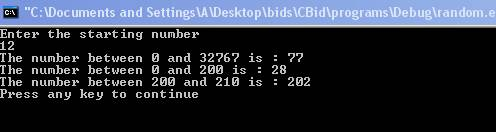Random Function

There are functions which are used to generate pseudo random numbers. In order to use these function header file <cstdlib> is included into the program. There are mainly two random functions in C++ such as rand() and  srand().

• rand () -  The function rand() generates the sequence of pseudo random numbers. It generates the random number between 0 and RAND_MAX.  RAND_MAX is a constant defined in header file <ctdlib>. A series of non related numbers are generated each time the function is called.

• srand() - The function srand()  uses the seed parameter  to set the starting point for the sequence of random numbers generated by the function rand().The parameter seed is unsigned integer. It is used to generate different sequences of pseudo random numbers by specifying different seed each time when the program is run. If the seed is not changed then the same sequence will be generated as pervious sequence when the program is run.

Here is a program which illustrates the working of random functions.

#include<iostream>

#include<cstdlib>

using namespace std;

int main()

{

int a,b,c,d;

cout << "Enter the starting number" << endl;

cin >> a;

srand(a);

b=rand()%RAND_MAX;

c=rand()%200;

d=rand()%10+200;

cout << "The number between 0 and " << RAND_MAX  << " is : " << b << endl;

cout << "The number between 0 and 10 is : " << c << endl;

cout << "The number between 200 and 210 is : " << d  << endl;

return(0);

}

The result of the program is:-The statement

#include<cstdlib>

I

includes  a header file <cstdlib> into the program. The statement

srand(a);

sets the starting point of the sequence of random numbers to the value of the variable a. The value entered by the user is 12. The statement

b=rand()%RAND_MAX

returns the number between 0 and RAND_MAX. The statement

c=rand()%200;

returns the random number between 0 and 200. The random number returned is 28. The statement

d=rand()%10+200;

returns the random number between 200 and 210. The number returned is 202. The statement

cout << "The number between 0 and " << RAND_MAX  << " is : " << b << endl;

displays the value of RAND_MAX and random number between 0 and RAND_MAX which is 77. . The value of RAND_MAX is 32767.

Go to the previous lesson or proceed to the next lesson